0
5674

# Visual Reasoning Questions for MBA CET PDF

Download MAH MBA CET Visual Reasoning (Abstract Reasoning) Questions and Answers PDF covering the important questions. Most expected visual reasoning questions with explanations for MAH MBA CET / MMS CET 2021 exam.

Question 1: Four positions of a dice are given below: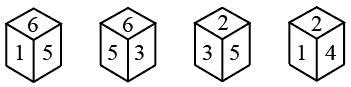Find the number on the face opposite to the face showing 4.

a) 5

b) 3

c) 6

d) 1

Question 2: Which answer figure will complete the pattern in the question figure?
Question Figure: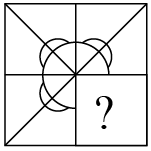a)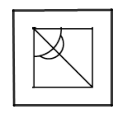b)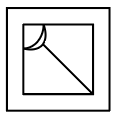c)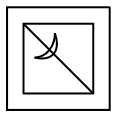d)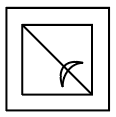Question 3: If a mirror is placed on the line MN, then which of the answer figures is the right image of the given figure?a)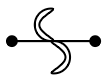b)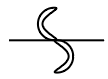c)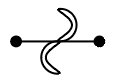d)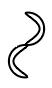Question 4: In the following question, select the figure which can be placed at the sign of question mark (?) from the given alternatives.

a)

b)

c)

d)

Question 5: From the given answer figures, select the one in which the question figure is hidden/embedded.

a)

b)

c)

d)

Question 6: Select the figure that will come next in the following series.

a)

b)

c)

d)

Question 7: Study the given pattern carefully and select the figure that will complete the pattern given in the question figure.

a)

b)

c)

d)

Question 8: Select the correct mirror image of the given figure when the mirror is placed at line AB.

a)

b)

c)

d)

Question 9: How many triangles are present in the following figure ?

a) 11

b) 9

c) 10

d) 13

Question 10: A paper is folded and cut as shown in the following problem figures (X, Y and Z). Select the answer figure that depicts how the paper will appear when unfolded.

a)

b)

c)

d)

Question 11: A figure of transparent sheet with a pattern is given below. Select the option that shows how the pattern would appear when the transparent sheet is folded at the dotted line.

a)

b)

c)

d)

Question 12: Select the correct mirror image of the given figure when the mirror is placed to the right of the figure.

a)

b)

c)

d)

Question 13: choose the Alternatives the figure the best that completes the pattern given below?

a)

b)

c)

d)

Question 14: Select the option that will correctly fit in the blank space in the given figure series.

a)

b)

c)

d)

Question 15: Look carefully at the sequence of symbols to find the pattern.

Which of the following will replace the (?) in the sequence?

a)

b)

c)

d)

Question 16: Out of the four option figures, three are similar in a certain manner. However, one figure is NOT like the other three.
Select the figure which is different from the rest.

a) C

b) B

c) D

d) A

Question 17: Find the ODD one:

a) b

b) d

c) a

d) c

Question 18: Find the next figure for the given series:

a) A

b) D

c) B

d) C

Question 19: Which pattern will the given transparent sheet resemble when it is folded at the dotted line?

a) C

b) B

c) A

d) D

Question 20: Select the option that can replace the question mark (?) in the following series.

a) C

b) D

c) A

d) B

From the first position of dice, 5 is not opposite to 1 and 6

From the third position of dice , 5 is not opposite to 2 and 3

$\Rightarrow$ 5 is opposite to the remaining number 4

$\therefore\$5 is the number on the face opposite to the face showing 4

Hence, the correct answer is Option A

The figure which completes the question figure is similar to the remaining three parts of the question figure.

$\therefore\$The figure will complete the pattern in the question figure is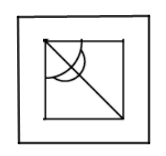Hence, the correct answer is Option A

When a mirror is placed on the line MN,

The facing of the figure to the left and right direction changes to opposite direction in mirror image(right facing changes to left and vice versa). There won’t be change in shape of the mirror image.

$\therefore\$The mirror image of the given figure is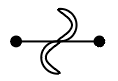Hence, the correct answer is Option C

From the first figure it is known that the empty circle and triangle are inserted inside the square.

In second figure, filled circle and triangle are inserted over the empty circle and triangle.

In the third figure, another empty circle and triangle are inserted.

From the fifth diagram we can see that another empty empty circle and triangle must be inserted in the fourth figure.

Therefore option C is the answer.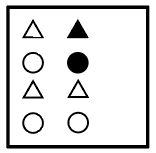The answer would be option b.

Here the letters are moving 90 degree clockwise direction so, the answer figure will be option C.

A vertical mirror is placed, so the object on the left will appear on the right and vice versa.

Thus, the three horizontal lines at top right in question figure will now appear at top left, as shown only in first figure. (Note: order of the lines will not be changed, i.e. the smallest will still remain at the bottom)

=> Ans – (A)

In top of the figure 3 traingles is there 2 small and 1 big

In bottom of the figure 3 triangles is there 2 small and 1 big

So total = 3 + 3 = 6

Now in middle of the figure 3 small and 2 big triangles is there = 3+2=5

Total triangles = 6+5=11

If we do by using practical paper and follow from the last figure then we observes that figure of option D will appear when unfolded.

If we assume the dotted line as a mirror then according to the law of mirror image the nearest one to the mirror will remain the nearest one after formation of the mirror image. So, here option D is the right one.

If we place the mirror to the right side then the letter or number which is nearest to the mirror will be nearest after the formation of mirror image and the letters and numbers are changed accordingly.

When we replace the square containing question mark with the options one bye one, Option A alone best suits the square as it represents the same pattern followed in the question.

Replacing it with Option A:

Square 3 and 4 have $+$ inside the circle and $O$ outside the circle.In the same way Squares 1 and 2 must have $O$ inside the circle and $+$ outside the circle.

The pattern is :

First  semi-circle is decreased.

and in the next figure is decreased.

So , the next figure would be

So , answer would be option b.

If we observe the pattern then we see that the elements star and triangle at the top and bottom interchange their places, and all the rest of the elements move anti clock wise.

All figure contain same as itself except figure D(hexagon contain quadilateral),

Hence option ‘C’ is correct.

In figure D oval shape is present and other figure have line or circle ,

Hence option ‘B’ is correct.

B is the missing option as it has a inverted question mark shaped symbol over it same as that of the 2nd figure in the question.

Option C is correct.